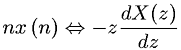Equations > Signal Processing > Z Transform Properties > Z-transform multiplication by a ramp (z domain differentiation) property

### Z-transform multiplication by a ramp (z domain differentiation) propertyLatex Code:

MathML Code:

 $\mathrm{nx}\left(n\right)⇔-z\frac\mathrm{dX}\left(z\right)\mathrm{dz}$

MathType 5.0: